The First law of thermodynamics is a statement of law of conservation of energy. Also, according to this law, heat and work are interchangeable. Any system that violates the first law (i.e., creates or destroys energy) is known as a Perpetual Motion Machine (PMM) of first kind.

For a system undergoing a cyclic process, the first law of thermodynamics is given by:This Equations can be Written as: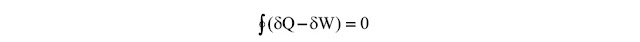This implies that (δW-δQ) must be a point function or property of the system. This property is termed as internal energy, U. Mathematically, internal energy can be written as: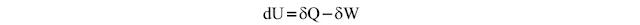The internal energy of a system represents a sum total of all forms of energy viz. thermal, molecular, lattice, nuclear, rotational, vibrational etc.

First law of thermodynamics for a closed system:

Let the internal energy of a closed system at an equilibrium state 1 be U1. If 1Qamount of heat is transferred across its boundary and 1Wis the amount of work done by the system and the system is allowed to come to an equilibrium state 2. Then integration of above Equation yields,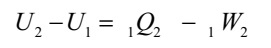If m is the mass of the system and u denotes the specific internal energy of the system then,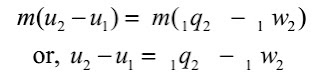where, 1q2 and 1ware heat transfer and work done per unit mass of the system.

Flow Work:

In an open system some matter, usually fluid enters and leaves the system. It requires flow work for the fluid to enter the system against the system pressure and at the same time flow work is required to expel the fluid from the system. It can be shown that the specific flow work is given by the product of pressure, p and specific volume, v, i.e.,

Flow work = pv.

Enthalpy:

In the analysis of open systems, it is convenient to combine the specific flow work ‘pv’ with internal energy ‘u’ as both of them increase the energy of the system. The sum of specific internal energy and specific flow work is an intensive property of the system and is called specific enthalpy, h. Thus specific enthalpy, h is given by: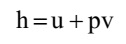First law of thermodynamics for open system:

For an open system shown in Figure, m1 and m2 are the mass flow rates at inlet and outlet, h1 and h2 are the specific enthalpies at inlet and outlet, V1 and V2 are the inlet and outlet velocities and z1 and z2 are the heights at inlet and outlet with reference to a datum; q and w are the rate of heat and work transfer to the system and E is the total energy of the system.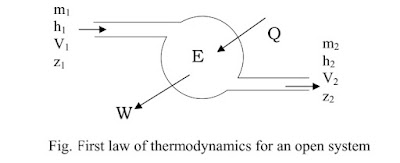Then the first law for this open system is given by: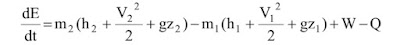where (dE/dt) is the rate at which the total energy of the system changes and ‘g’ is the acceleration due to gravity.

First law for open system in steady state:

In steady state process, the time rate of change of all the quantities is zero, and mass is also conserved. As a result, the mass and total energy of the system do not change with time, hence, (dE/dt) is zero and from conservation of mass, m1 = m2 = m. Then the first law becomes: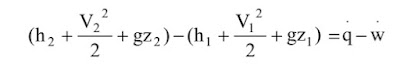Where, q and w are specific heat and work transfer rates.